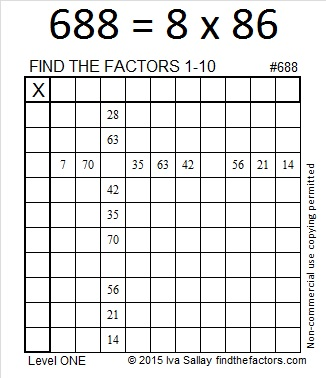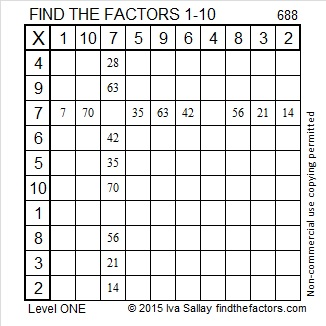# 688 is a Friedman number

688 is a palindrome in two different bases:

• 2002 in base 7; note that 2(343) + 0(49) + 0(7) + 2(1) = 688
• 494 in base 12; note that 4(144) + 9(12) + 4(1) = 688

688 is called a Friedman number because it can be expressed using only its own digits and +, -, x, ÷, ( ), and exponents. 688 = 8 x 86 so I made a factor tree based on that single multiplication fact:Since it’s such a fun number fact, I positioned it on top of today’s factoring puzzle, too.Print the puzzles or type the solution on this excel file: 10 Factors 2015-11-23

—————————————————————————————————

• 688 is a composite number.
• Prime factorization: 688 = 2 x 2 x 2 x 2 x 43, which can be written 688 = 2⁴ x 43
• The exponents in the prime factorization are 4 and 1. Adding one to each and multiplying we get (4 + 1)(1 + 1) = 5 x 2 = 10. Therefore 688 has exactly 10 factors.
• Factors of 688: 1, 2, 4, 8, 16, 43, 86, 172, 344, 688
• Factor pairs: 688 = 1 x 688, 2 x 344, 4 x 172, 8 x 86, or 16 x 43
• Taking the factor pair with the largest square number factor, we get √688 = (√16)(√43) = 4√43 ≈ 26.229754.—————————————————————————————————